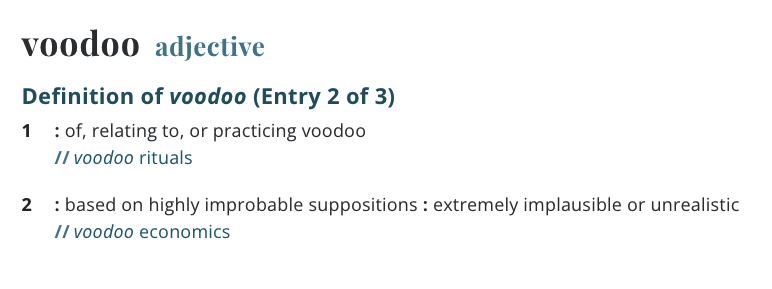IF - IG
International Interdisciplinary Center For Information and Fact Checking about Info-Gap Decision TheoryThe rise and rise of voodoo decision making### What exactly is Info-Gap Decision Theory (IGDT)?

This is an interesting, and extremely relevant question to our project here at IF-IG. The exact and definitive answer to this simple question is that ... it all depends! That is, it all depends on the person to whom you pose this question.

According to advocates and promoters of this theory, IGDT is a relative new decision theory that seeks robust solutions to decision-making situations that are subject to severe uncertainty.

In contrast, according to some critics, IGDT is a misapplication of the well established concept Radius of Stability that is utterly unsuitable for the treatment of severe uncertainty of the type assumed by IGDT. Some go so far as to claim that IGDT is a voodoo decision theory par excellence.

The main goal of the IF-IG project is to provide a clear answer to the above question and to explain why IGDT is in fact fundamentally flawed.

Since the term "voodoo" has various meanings, it is important to point out that in this discussion this term has the meaning it takes in phrases like "voodoo economics", "voodoo science", "voodoo mathematics", and so on. Observe that in the Merriam-Webster dictionary we read:So, in this discussion, the term voodoo decision theory is used to describe a theory that is based on extremely unrealist, perhaps even contradictory, assumptions.

Back to IGDT.

To explain what IGDT is all about, it is instructive to compare it to the well known and well established Wald's maximin paradigm.

 Wald's maximin paradigm IGDT (Robust) decision model The best decision is one whose security level is at least as good (large) as the security levels of all other decisions under consideration. The best decision is one whose radius of stability is at least as good (large) as the radii of stability of all other decisions.

So, at this level of abstraction we can say that

 IGDT Robust decision model is a special case of Wald's maximin paradigm where the security level of a decision is defined as its radius of stability.

So, now we have to explain the meaning of the 'intuitive' concept Radius of Stability. There are numerous formal and informal definition of this fundamental concept. For our purposes here, it suffices to say this: consider the situation depicted in this figure:where

• S = region of stability of parameter p,

• I = region of instability of parameter p,

and the center of the circles is the nominal value of p under consideration.

In this context we have:

 The radius of stability of a system at a given nominal state is the radius of the largest ball centered at the nominal state, all of whose elements are stable. Equivalently, it is the size of the smallest perturbation in the nominal state that destabilizes the system. The larger the radius of stability, the more stable (robust) the system is to small perturbations in the nominal state.

In short,

 The radius of stability of a system at a given nominal state is the longest distance we can move (in a worst-case sense*) from the nominal state without destabilizing the system.

* In all direction.

 The radius of stability of a system at a given nominal state is the radius of the largest ball centered at the nominal state, all of whose elements are stable. Equivalently, it is the size of the smallest perturbation in the nominal state that destabilizes the system. The larger the radius of stability, the more stable (robust) the system is to small perturbations in the nominal state.

For the record, it should be emphasized that the use of the concept Radius of Stability dates back to the 1960s. IGDT reinvented this concept in 2001 and called it info-gap robustness. Furthermore, not only that for years advocates of IGDT have ignored the fact that info-gap robustness is a reinvention of the concept Radius of Stability, the theory was originally presented as "new" and "radically different" from the theories available at that time!

 Info-gap decision theory is radically different from all current theories of decision under uncertainty. The difference originates in the modeling of uncertainty as an information gap rather than as a probability. Ben-Haim (2001, 2006, p. xii)

It should be stressed that there is noting wrong in using the concept Radius of Stability as a measure of robustness or stability under appropriate conditions. The trouble with IGDT is that it misapplies this fundamental, intuitive, and useful concept, namely it applies it in situations where this measure of robustness/stability does not address the core problem the theory is designed to solve. In particular, the Radius of Stability is not an appropriate tool for measuring robustness/stability in the framework of the severe uncertainty stipulated by IGDT. In other words, this measure is incompatible with the severity of the uncertainty under consideration.

More technically speaking, the analysis of the severe uncertainty stipulated by IGDT requires a global robustness analysis. Instead IGDT administer a local robustness analysis based on a Radius of stability model. By analogy, it is akin to administering local anesthetic instead of the required general (i.e., global) one.

You can find plenty of information about IGDT in the old site, right here.